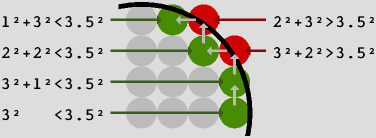# CircleFill Algorithm

by Alain Brobecker (aka baah/Arm's Tech)
(abrobecker@yahoo.com or rte de Dardagny; 01630 CHALLEX; FRANCE).

The idea of using (dx+1)2=dx2+2*dx+1 to track circle's edge was given by Frederic Elisei (ArmOric/Arm's Tech), and i got the threshold% optimisation from the circle routine of Jan Vlietinck (Jan/BASS). All the rest (clipping, using r+0.5) was devised by me.

### CircleFill

Before going on let's have some physical modelisation: the screen is composed of picture cells (pixels) we'll assimilate to small circles, and the integer coordinates (x;y) of a pixel will refer to its center where the electron beam hits the screen, luminosity then decreasing with the distance (thus explaining the circular model).

A circle of center (x0;y0) and of radius r is the set of points (x;y) so that dx2+dy2<r2, where dx=x-x0 and dy=y-y0. We'll use this definition with a slight modification, using (r+1/2)2 instead of r2, because it gives better results. Let's have an example, where the center (x0;y0) is the bottom-left pixel, and the radius is 3.We start at scanline y0 by drawing the segment between x0-3 and x0+3 (green pixel at the bottom). Then we go up one line and compute the new distance between the center and (x0+3;y0+1), it is still lower than (r+1/2)2 so this pixel is an edge for scanline y0+1 (the other one is at x0-3). Go up one more line, but this time the distance to (x0+3;y0+2) is too big. So we go left one pixel, and since the distance to (x0+2;y0+2) is lower than (r+1/2)2 anew, this is an edge for this scanline...

We notice that when we know the distance between the center and a pixel, we can compute the one between the center and the 4 adjacent pixels with additions only (eg dx2+(dy+1)2=dx2+dy2+2*dy+1). So we'll use this to track the edges of the circle, beginning at (x0+r;y0). The algorithm looks like this:

```    REMUnclipped CircleFill by Alain Brobecker (aka baah/Arm's Tech)
DEFPROCcirclefill(x%,y%,r%)
dx%=r%
IFdx%=0THENENDPROC
dist%=r%*r%
distmax%=dist%+r%             :REMshould be (r%+0.5)^2=r%^2+r%+0.25
LINEx%-dx%,y%,x%+dx%,y%       :REMdraw central line
dist%+=1
dy%=1
WHILEdy%<=r%
WHILEdist%>distmax%
dist%+=-2*dx%+1           :REMdist%=(dx%-1)^2+dy^2=dist%-2*dx%+1
dx%-=1
ENDWHILE
LINEx%-dx%,y%+dy%,x%+dx%,y%+dy% :REMdraw double lines
LINEx%-dx%,y%-dy%,x%+dx%,y%-dy%
dist%+=2*dy%+1              :REMdist%=dx%^2+(dy+1)^2=dist%+2*dy%+1
dy%+=1
ENDWHILE
ENDPROC
```

We can optimise by noticing that only the sign of threshold%=dist%-distmax% interests us, so we don't need to compute r%*r%, we spare a register and the while can be implemented more cunningly in assembly. Jan also did use more symmetries since he didn't clip the circle, having four lines drawn and at most one correction with the dx term per iteration.

### Clipped Version

The horizontal clipping will be performed during the line drawing. Of course if we draw symmetrical lines at position y0+dy and y0-dy, this clipping needs to be made once only. For vertical clipping, the important note is that we can know beforehand how the circle will be clipped:

1. if y0 is out of the screen, we begin to compute the edges without drawing, and once y0+dy is in the screen we draw single lines only.
2. if y0 is in the screen, we draw double lines until the top or the bottom of the circle goes out of the screen (if this happens at all) and then draw single lines only (same routine as case 1). To know which side (top or bottom) is clipped first, we check if y0<screen_height/2 or not.

The algorithm below may seem complex since it comes from the assembly version (which was written first =), and the GOTOs may frighten you, but many parts are duplicated, so it is not that big. The variables nbd% and nbs% are the number of double and single lines to draw.

``` 1 : MODE9:ORIGIN0,256:OFF:scrx%=320:scry%=128
2 : REPEAT
3 :   GCOL3,1
4 :   x%=RND(480)-80
5 :   y%=RND(316)-80
6 :   r%=RND(120)
7 :   PROCcirclefill(x%,y%,r%):CIRCLEFILLx%<<2,y%<<2,r%<<2
8 : UNTIL0
9 :
10 : REMCircleFill by Alain Brobecker (aka baah/Arm's Tech)
11 : DEFPROCcirclefill(x%,y%,r%)
12 :   IFr%<0THENENDPROC
13 :   IF(x%+r%<0)OR(y%+r%<0)THENENDPROC
14 :   IF(x%-r%>=scrx%)OR(y%-r%>=scry%)THENENDPROC
15 :   dx%=r%
16 :   inc%=1              :REMscrx for an assembly version
17 :   IFy%<0THENGOTO 60   :REMSkipU2D
18 :   IFy%>=scry%THENGOTO 77 :REMSkipD2U
19 :   LINE x%-dx%<<2,y%<<2,x%+dx%<<2,y%<<2
20 :   IFy%<scry%/2THEN
21 :     nbd%=y%
22 :   ELSE
23 :     inc%=-inc%
24 :     nbd%=scry%-1-y%
25 :   ENDIF
26 :   y1%=y%+inc%
27 :   y2%=y%-inc%
28 :   IFr%<nbd%THENnbd%=r%
29 :   nbs%=r%-nbd%
30 :   IFscry%-(2*nbd%+1)<nbs%THENnbs%=scry%-(2*nbd%+1)
31 :   threshold%=r%-1
32 :   dy%=1
33 :   WHILEnbd%<>0
34 :     WHILEthreshold%<0
35 :       threshold%+=2*dx%-1
36 :       dx%-=1
37 :     ENDWHILE
38 :     LINEx%-dx%<<2,y1%<<2,x%+dx%<<2,y1%<<2
39 :     LINEx%-dx%<<2,y2%<<2,x%+dx%<<2,y2%<<2
40 :     y1%+=inc%
41 :     y2%-=inc%
42 :     threshold%-=2*dy%+1
43 :     dy%+=1
44 :     nbd%-=1
45 :   ENDWHILE
46 : REMdraw the single lines
47 :   WHILEnbs%<>0
48 :     WHILEthreshold%<0
49 :       threshold%+=2*dx%-1
50 :       dx%-=1
51 :     ENDWHILE
52 :     LINEx%-dx%<<2,y1%<<2,x%+dx%<<2,y1%<<2
53 :     y1%+=inc%
54 :     threshold%-=2*dy%+1
55 :     dy%+=1
56 :     nbs%-=1
57 :   ENDWHILE
58 : ENDPROC
59 :
60 : REMy<0 so skip -y lines by computing -y-1 times the loop without drawing
61 :   y1%=0
62 :   nbs%=y%+r%+1:IFnbs%>scry%THENnbs%=scry%
63 :   threshold%=r%-1
64 :   dy%=1
65 :   WHILEy%<>-1
66 :     WHILEthreshold%<0
67 :       threshold%+=2*dx%-1
68 :       dx%-=1
69 :     ENDWHILE
70 :     threshold%-=2*dy%+1
71 :     dy%+=1
72 :     y%+=1
73 :   ENDWHILE
74 :   GOTO 46
75 :
76 : REMy>scry so skip y-scry lines
77 :   y1%=scry%-1
78 :   nbs%=scry%-(y%-r%):IFnbs%>scry%THENnbs%=scry%
79 :   inc%=-inc%
80 :   threshold%=r%-1
81 :   dy%=1
82 :   WHILEy%>scry%
83 :     WHILEthreshold%<0
84 :       threshold%+=2*dx%-1
85 :       dx%-=1
86 :     ENDWHILE
87 :     threshold%-=2*dy%+1
88 :     dy%+=1
89 :     y%-=1
90 :   ENDWHILE
91 :   GOTO 46
```